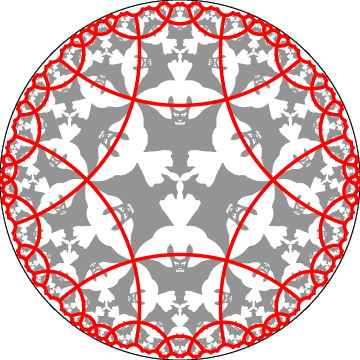## 1. Introduction

M. C. Escher created many repeating patterns of the Euclidean plane. In a few cases he distorted or transformed these patterns to obtain new patterns in other geometries: spherical or hyperbolic. Escher's Euclidean Notebook Pattern Number 45 of angels and devils is the only one that he converted to both the sphere and the hyperbolic plane. These three related patterns are shown in (Schattschneider 1990) on pages 150, 244, and 296; see Figure 1 below for a rendition of Circle Limit IV. Professor Coxeter discusses the three patterns on pages 197-209 of (Coxeter 1981).Figure 1: A rendition of Escher's hyperbolic pattern Circle Limit IV of angels (the white background) and devils (foreground).

There are probably many ways to distort or transform one pattern into another. The method we will describe applies to repeating patterns based on the regular tessellations, {p,q}, composed of regular p-sided polygons meeting q at a vertex. Thus, given one repeating pattern, we could theoretically create a doubly infinite family of related patterns by transforming the original pattern into others based on different values of p and q. Many of Escher's Euclidean patterns and all of his spherical and hyperbolic patterns are based on {p,q}. For example, his Euclidean Notebook Pattern Number 45 and the related spherical and hyperbolic patterns mentioned above are based on the tessellations {4,4}, {4,3}, and {6,4} respectively. Figure 2 below shows the tessellation {6,4} superimposed on the Circle Limit IV pattern. In these patterns, p is twice the number of angels/devils that meet at their feet and q is the number of wing tips that meet at a point. The meeting point of feet is the intersection of lines of bilateral (reflection) symmetry - hence the need to double the number of angels/devils to obtain p.Figure 2: The Circle Limit IV pattern showing the underlying {6,4} tessellation.

We will begin with a brief review of hyperbolic geometry. Then we discuss repeating patterns and regular tessellations, and the transformation process, showing an example. Next we will show other examples based on Escher's four hyperbolic patterns. Finally we suggest possible further directions of research.

 INDEX NEXT